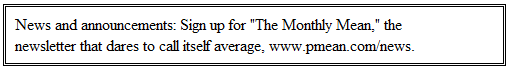P.Mean: Poem to help you remember the quotient rule (created 2010-11-26).I was working on some derivatives then involved a fraction, and the formula is a bit tricky to remember. There was a short poem that I learned a long time ago for the derivative of a fraction, and I can't find it anywhere on the Internet. There are some variants that are close, but nothing quite like the poem I remember. Everything important has to be found somewhere on the Internet, so I am posting the poem here. If anyone can attribute this poem to the original source, please let me know.

If quotient rule you wish to know,
It's lo d hi less hi d lo.
Draw the line and down below
Denominator squared will go.

There's a variation like this based on the Old McDonald song,

Lo-de-hi less hi-de-lo EIEIO
Then draw the line and down below EIEIO
With a dx here and a dy there Here a slope, yes there's hope, you can cope
Denominator squared will go EIEIO

-- http://www.xs4all.nl/~jcdverha/scijokes/11_2.html

but it's not nearly as good as the version I remember.

Here's the algebraic version:Here's how it corresponds to the poem:

• hi = g(x)
• lo = h(x)

So

• lo d hi = h(x) g'(x)
• hi d lo = g(x) h'(x)

Here's the recent example I used it on.The derivative of exp(x) is exp(x) and the derivative of 1+exp(x) is exp(x). So there is a lot of repetition going on here. But see if you can follow the logic.For the record, this simplifies toThis work is licensed under a Creative Commons Attribution 3.0 United States License. This page was written by Steve Simon and was last modified on 2017-06-15. Need more information? I have a page with general help resources. You can also browse for pages similar to this one at Incomplete pages.